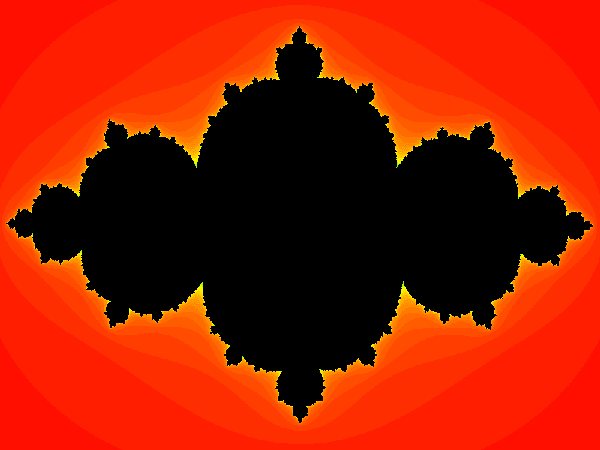## Posts

Showing posts from May, 2016

### Image edge detectionEdge detection  is a fundamental tool in image processing, machine vision and computer vision. In this post we're going to take a look at a very basic edge detection algorithm, which takes into account a predefined tolerance value that can be adjusted to detect arbitrary fine details in a given image. # Algorithm The algorithm is extremely simple; it begins by iterating over each pixel in the image, then, for each pixel, it checks its neighbors. +-------+-------+-------+ |       |       |       | |   A   |   B   |   C   | |       |       |       | +-------+-------+-------+ |       |         |       | |   D   |         |   E   | |       |         |       | +-------+-------+-------+ |       |       |       | |   F   |   G   |   H   | |       |       |       | +-------+-------+-------+ If any two opposite neighbors differ in intensity by more than a predefined tolerance, then it marks the current pixel as part of an edge. The comparison is done by taking th

### Julia setThe Julia set and its complex beauty. A complex system in the Julia set can be defined as simply as: z = z^2 + c where both z and c are complex numbers. # Algorithm The value of z is updated iteratively for a predefined number of times (e.g.: 100) or while the absolute value of z is lower than a given constant (e.g. \abs(z) < 2). The initial value of z is set as: z = Complex ( ( 2 * x - w ) / ( w * zoom ) + moveX , ( 2 * y - h ) / ( h * zoom ) + moveY ) where x and y are the coordinate positions on a 2D plane, w and h are the width and height of the plane.  The values for "zoom", "moveX" and "moveY" are optional. They control the level of zoom into the fractal and the offsets for shifting the image on the X and Y axis. The iteration loop can be defined as: i = 100 # maximum number of iterations while ( abs ( z ) < 2 && -- i > 0

### The beauty of InfinityInfinity and its infinite beauty. Today we're going to take a brief look at the beauty of infinity, through: infinite sums infinite products continued fractions nested radicals and limits to represent some of the most well known and beautiful mathematical constants, like  π , e and φ . # Definitions Before diving into it, let's begin with some simple definitions: 1) Infinite sum: \sum_{n=1}^(\infty)\frac{1}{n} = \frac{1}{1} + \frac{1}{2} + \frac{1}{3} + \... 2) Infinite product: \prod_{n=1}^(\infty)\frac{1}{n} = \frac{1}{1} * \frac{1}{2} * \frac{1}{3} * \... 3) Continued fractions: \underset{n=1}{\overset{\infty}{\mathbf{K}}}\frac{1}{n} = \frac{1}{1 + \frac{1}{2 + \frac{1}{3 + \...}}} 4) Nested radicals: \underset{n=1}{\overset{\infty}{\mathbf{R}}}(n + R)^(1/2) = \sqrt{1 + \sqrt{2 + \sqrt{3 + \...}}} 5) Limit: \lim_{n to \infty}(1 + 1/n)^n = (1 + 1/n)^n, where n approaches \infty. # Infinite sums `\sum_{n=0}^(\in

### MultisetsIn mathematics, a set is a collection of distinct objects, while a multiset  (or bag) is a generalization of the concept of a set, allowing multiple instances of a given object to exist in the same multiset. Working with multisets is a little bit more trickier and more computationally challenging, but it's quite fun, as we'll see in a moment. In programming, we can simulate a multiset using an array. The fun part comes when we have to implement some operations on this multisets, but we have to chose the right algorithms that will scale nicely and work efficiently even with multisets that contain more than a thousand elements. Before implementing the algorithms, let's take a brief look at some definitions of multiset operations. # Operations 1) Intersection (AND): The intersection of two multisets, A and B, denoted by A ∩ B, is the multiset of all things that are members of both A and B. [ 1 , 2 ] ∩ [ 2 , 3 ] = [ 2 ] [ 1 , 1 , 1 ,